Geodetector

measure and attribute stratified heterogeneity with software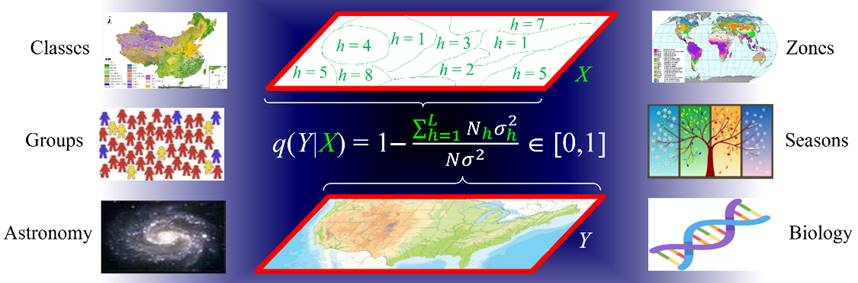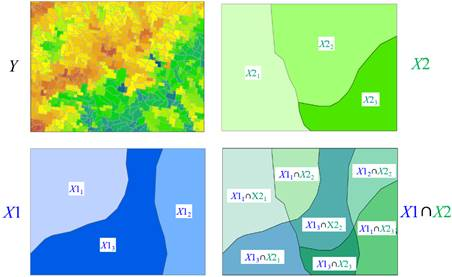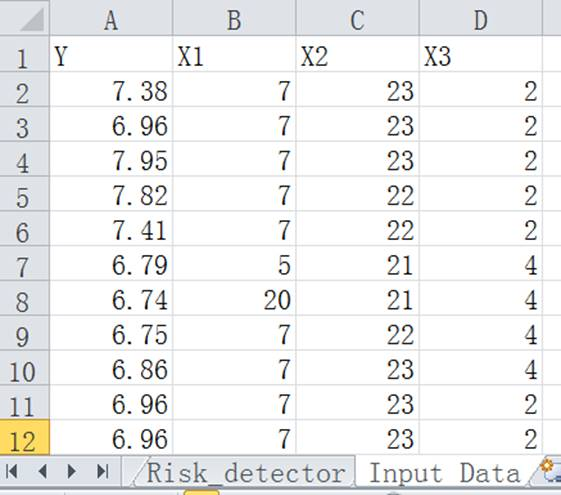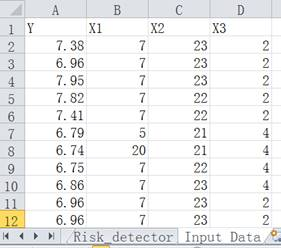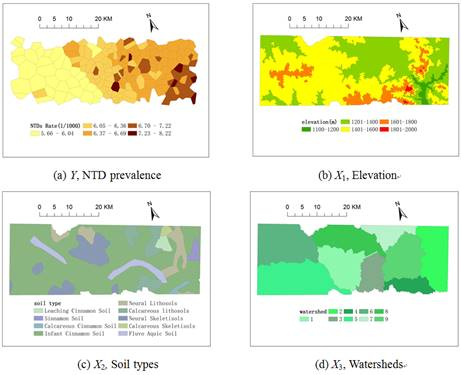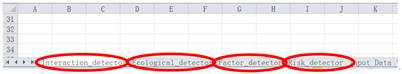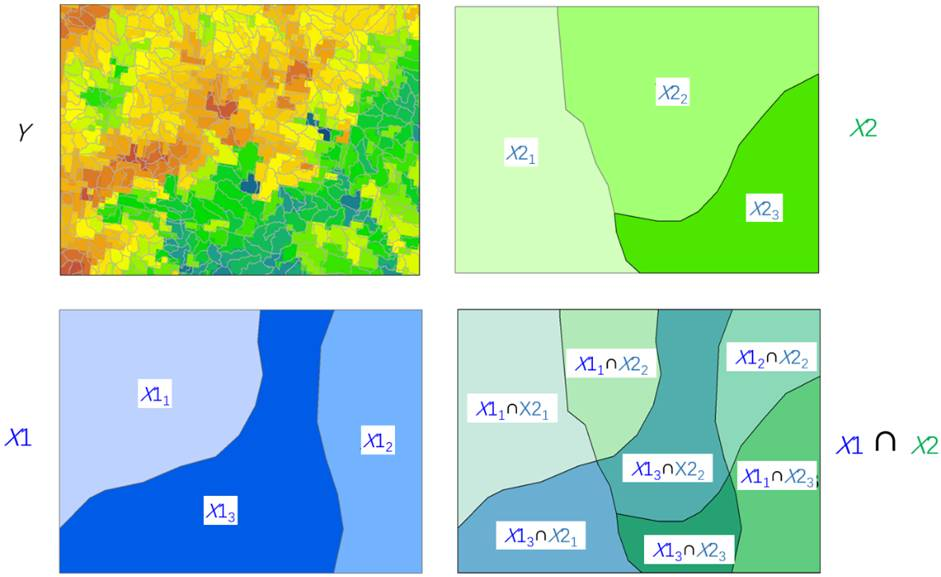Articles using Geodetector [Numbered]

 2024

Updated on 30 September 2023

# 1.        Introduction

Spatial Stratified Heterogeneity (SSH) is a phenomena that the within strata are more similar than the between strata. Examples of this include landuse types and climate zones in spatial data, seasons and years in time series, occupations, age groups, incomes strata. SSH occurs in all scales from universe to DNA, and has been studied since Aristotle time.

Geodetector, or Geographical Detector, is a statistical tool to measure SSH and to make attributions for or by SSH (Fig. 1):

(1) Measure and identity SSH among data;

(2) Test the coupling between two variables Y and X without assuming linearity of the association and with clear physical meanings; and

(3) Investigate the general interaction between two explanatory variables X1 and X2 and a response variable Y, without any specific form of interaction such as the assumed product in econometrics (Fig. 2).

Each of the above tasks can be accomplished using the Geodetector q-statistic: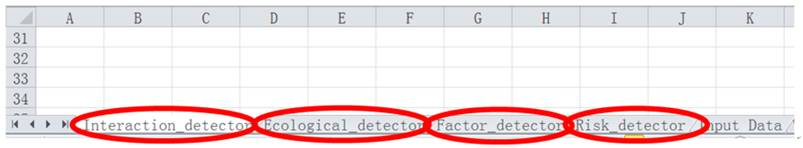Fig. 1. Principle of Geodetector q-statistic (Wang et al 2016)

(The bottom map, the color indicates the values of a population Y. The top map, the population Y is composed of L strata (h = 1, 2, ��, L); the terms ��stratification�� and ��partition�� are equivalent, can be either classification or zonation. Between the two maps is the equation q(Y|{h}), in which the numerator is the summation of the within strata variance and the denominator is the pooled variance; N and s2 stand for the number of units and the variance of Y in a study area, respectively. [(N-L)q]/[(L-1)(1-q)] ~ F(L-1, N-L, g), where g is a non central parameter)

.

The strata of Y (red polygons in Fig.1) are a partition of Y, either by Y itself or by an explanatory variable X. X is a categorical variable or should be stratified if it is a numerical variable. The number of strata L might be 2-10 or more, according to prior knowledge or a classification algorithm. The ��spatial�� in ��spatial stratified heterogeneity�� can be either spatial in geoscience or in a broad mathermatical sense such as time and any attributes.

Interpretation of Geodetector q-statistic (Fig.1).

The value of q is strictly within [0, 1].

(1)  If Y is stratified by Y itself, then a q-statistic of 0 indicates that Y is absent of spatial stratified heterogeneity; a q-statistic of 1 indicates that Y is perfectly spatially stratified heterogenous; and a q-statistic of 100q% measures the degree of spatial stratified heterogeneity of Y.

(2)  If Y is stratified by an explanatory variable X, then a q-statistic of 0 indicates that there is no coupling between Y and X; a q-statistic of 1 indicates that Y is completely determined by X; and X explains 100q% of Y. Please note that the q-statistic measures the association between X and Y without assuming the linearity between X and Y.

Geodetector q-statistic can be used to understand spatial confounding, sample bias and overfitting.

(1)    Confounding can occur if a model is applied to a (spatial) stratified heterogeneneous population, leading to a misleading interpretation and statistical insignificance of the model outcome. This problem can be avoided by identifying SSH (by Geodetector q statistic) then modelling in the strata, separately.

(2)    A sample would be biased if a population is (spatial) stratified heterogeneous and the sample do not cover all strata. The problem can be solved by identifing (spatial) stratified heterogeneity (using Geodetector q statistic) then applying bias remedy models such as Heckman regression and Bshade method.

(3)    Local models aim to overcome heterogeneity but often suffer from overfitting and too many parameters to interpret. These problems can be avoided by modelling in strata or stratifying the outputs of a local model then interpreting the stratified parameters.

Functions of Geodetector:

(1)    The risk detector maps response variable Y in strata according to X;

(2)    The factor detector q-statistic measures the degree of spatial stratified heterogeneity of a variable Y if Y is stratified by itself; and the determinant power of an explanatory variable X on Y if Y is stratified by X;

(3)    The ecological detector identifies the difference in the impacts between two explanatory variables X1 and X2;

(4)    The interaction detector reveals whether the risk factors X1 and X2 (and more X, if applicable) have an interactive influence on a response variable Y (Fig.2).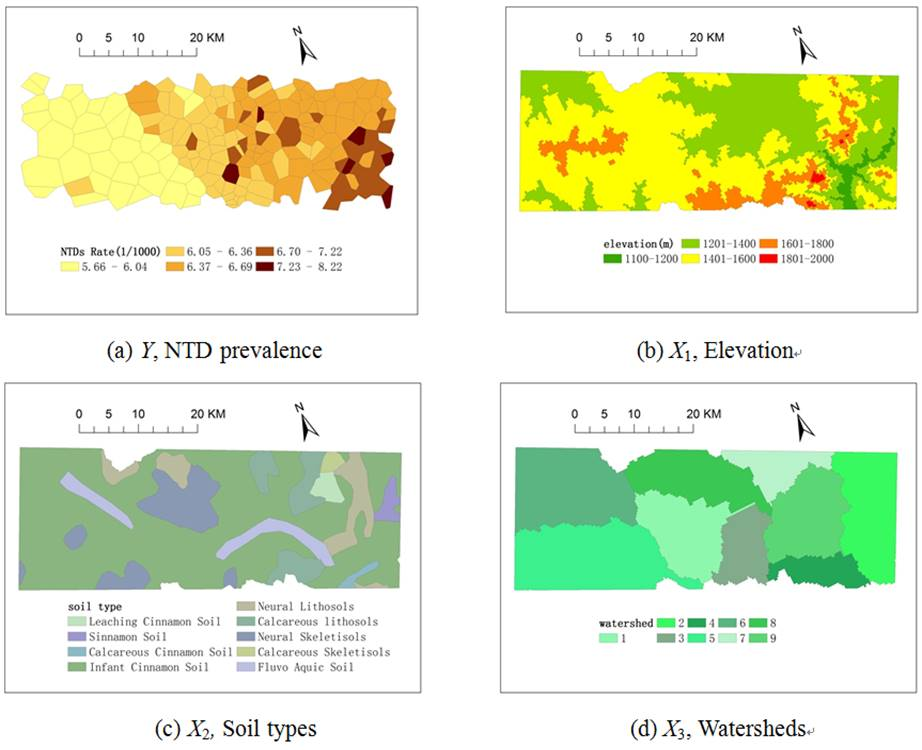Fig. 2. The General interaction between explanatory variables X1 and X2 impacting on a response variable Y: q(Y|X1X2).

# 2.       Tutorial

The Geodetector software was developed using Excel and R, respectively. The tools are free of charge, freely downloadable, and easy to use, and were designed without any GIS plug-in components and with ��one click�� execution. Users can run the following demo, then simply replace the demo data in the software using your own data, click Run and get results ! We henceforth describe Excel Geodetector software. R users can download the R Geodetector software in the following section ��Download of Geodetector Software and Example Datasets��.

As a demo, neural-tube birth defects (NTD) Y and suspected risk factors or their proxies Xs in villages are provided, including data for the health effect layers ��NTD prevalence�� and environmental factor layers, ��elevation��, ��soil type��, and ��watershed��. Their field names are defined as Y and X1, X2, X3 respectively.

(1)  Download the Excel Geodetector software (In the following section ��Software and Examples Data Download��), one click to download any one of the three Examples, unzip the downloaded file, you will find an Excel file (this is Geodetector software with an Example dataset!) and double click the Excel file, Fig. 3 and Fig. 5 appear. Fig. 3 is the format of the input data for the Geodetector: each row denotes a sample unit (e.g. a village); the 1st column record the response variable Y; the 2nd and following columns denote partitions of Y or factors X, the latter were partitioned according to the similarity within strata.

(2)  Input your data into the Excel Geodetector software in the format of Fig. 3. Then go to Step 2.Fig. 3. Input data in Excel and the execution interface

(Note: Y should be numerical; X MUST be categorical, e.g. landuse types, seasons. If X is numerical, it should be transformed into a categorical variable, e.g. GDP per capita is stratified into 5 strata. At lease three sample units in each of the strata are required)

(3)  If your data is in GIS format, as shown in Fig. 4, you can use QGIS directly in Section 4, or you can transform the GIS data into Excel data as shown in Fig. 3.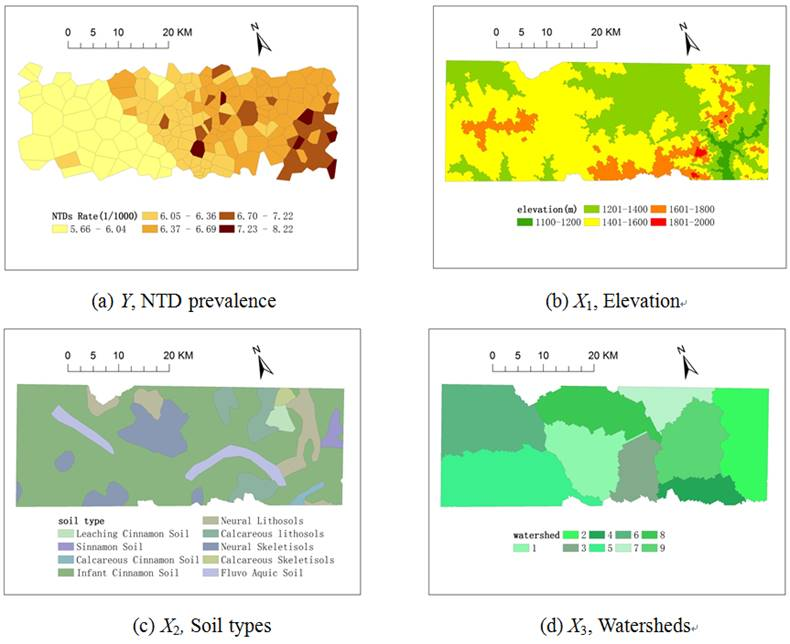Fig. 4. Data in GIS format

Step 2. Run Geodetector software

Only one operation interface was designed (Fig. 5). The function of the ��Read Data�� button is to load data; thus, when the button is clicked, all variables are listed in the ��variables�� list box. Then, disease and partition of Y or environmental factor variables are selected into their corresponding list boxes Y and X on the right of the interface. Finally, Geodetector is executed by clicking the ��Run�� button.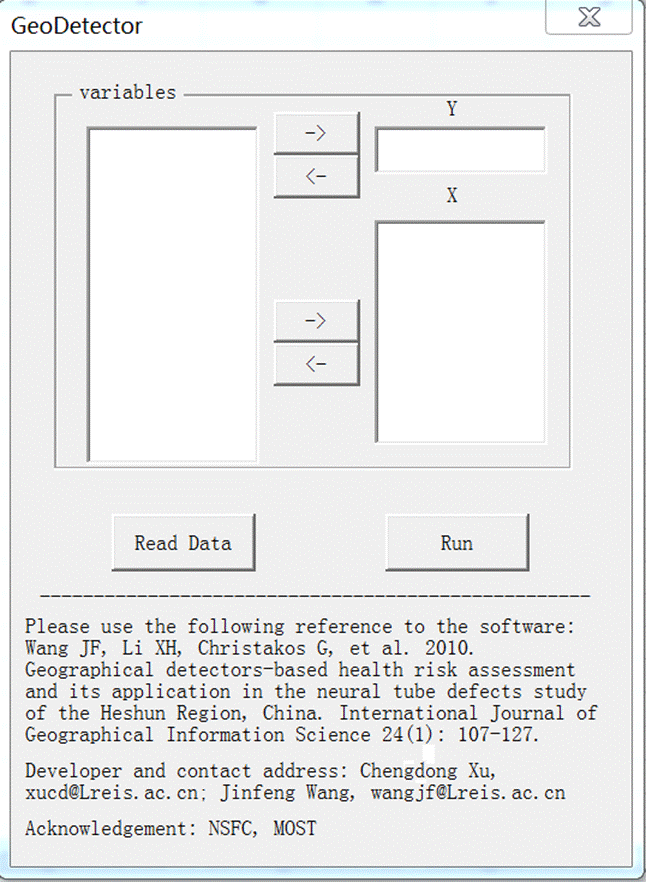Fig. 5. User interface for Geodetector

# 3.       Output

Geodetector outputs results from the risk detector, factor detector, ecological detector, and interaction detector in four Excel spreadsheets (Fig. 6).Fig. 6. Interface for Geodetector results

In the ��Risk detector�� sheet (Fig. 7), result information for each environmental risk factor is presented in two tables. The first table gives the average disease incidence in each stratum of a risk factor, the name of which is written at the top left of the table. The second table gives the statistically significant difference in the average disease incidence between two strata; if there is a significant difference, the corresponding value is ��Y��, else it is ��N��.Fig. 7. Results of risk detector

The Fig. 8 shows the output format of the q values for each environmental risk factor, as given in the ��Factor detector�� sheet. The table header gives the names of the environmental risk factors X(X1, X2, ��, Xn), while the associated q values and their corresponding p values are presented in the row below.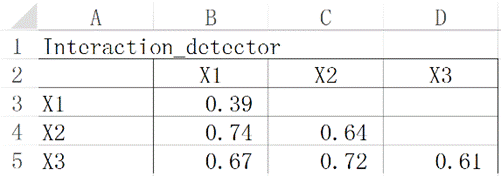Fig. 8. Results of factor detector

In the ��Ecological detector�� sheet (Fig. 9), results of the statistically significant differences between two environmental risk factors are presented. If Y(X1) (risk factor names in row) was significantly bigger than Y(X2) (risk factor names in column), the associated value is ��Y��, while ��N�� expresses the opposite meaning.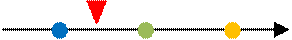Fig. 9. Results of ecological detector

The format of the results for the interaction detector is shown in Fig. 10. ��Interaction relationships�� below the table represent the interaction relationship for the two factors. The relationship is defined in a coordinate axis. It has 5 intervals, including ��(-����min(